# Year 5 Maths Printable Worksheets

Our year 5 maths worksheets are tailored to the Y5 maths curriculum and cover all the core maths topics including angles, percentages, mental maths, shapes and much more. Whether it's finding the difference between the area and perimeter of geometric shapes or adding and subtracting fractions, you will be sure to find a year 5 maths worksheet to suit your child's needs.

Filters

### Colour by number - Christmas decorations

This fun Christmas themed colour by number activity will help children recognise numbers 1 - 8. Children follow the number and colour chart to colour the Christmas tree and presents.

Age: 9 - 10
1
2
3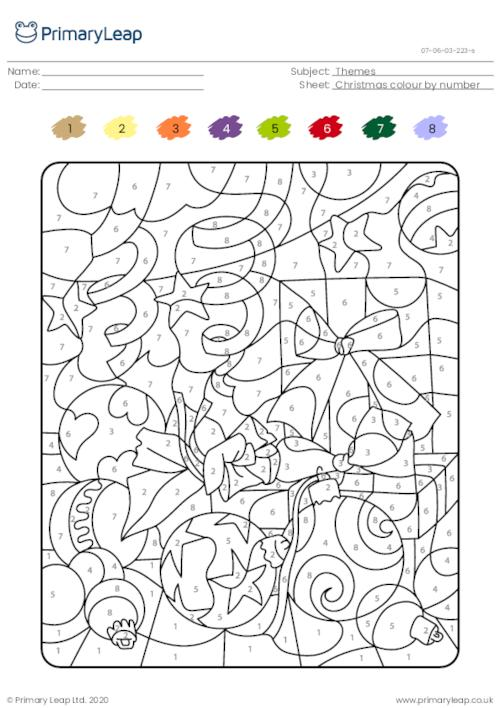### Christmas missing numbers 3

This Christmas-themed maths worksheet asks students to move in the direction of the arrow and write the correct number in the middle of each snowflake.

Age: 9 - 10
1
2
3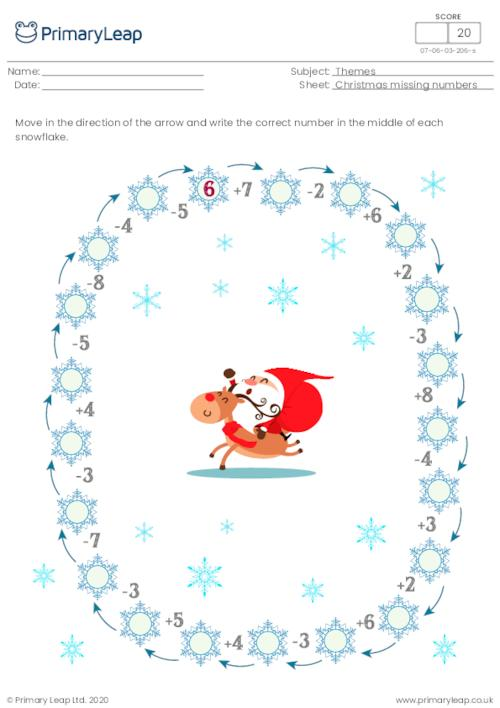### Christmas missing numbers 2

This Christmas-themed maths worksheet asks students to move in the direction of the arrow and write the correct number in the middle of each snowflake.

Age: 9 - 10
1
2
3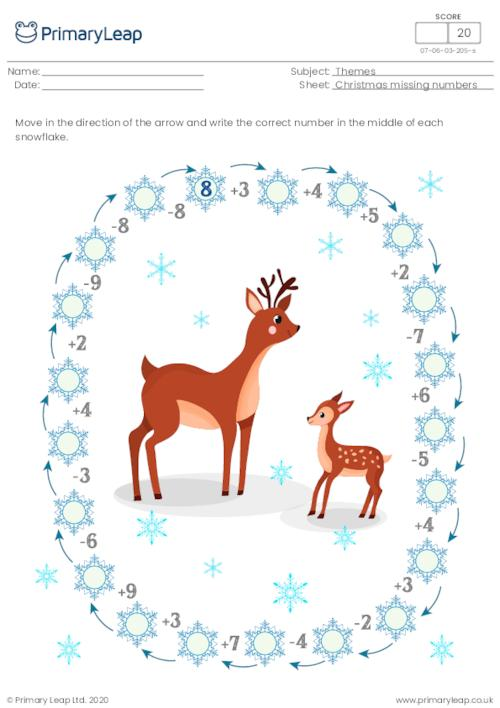### Christmas missing numbers 1

This Christmas-themed maths worksheet asks students to move in the direction of the arrow and write the correct number in the middle of each snowflake.

Age: 9 - 10
1
2
3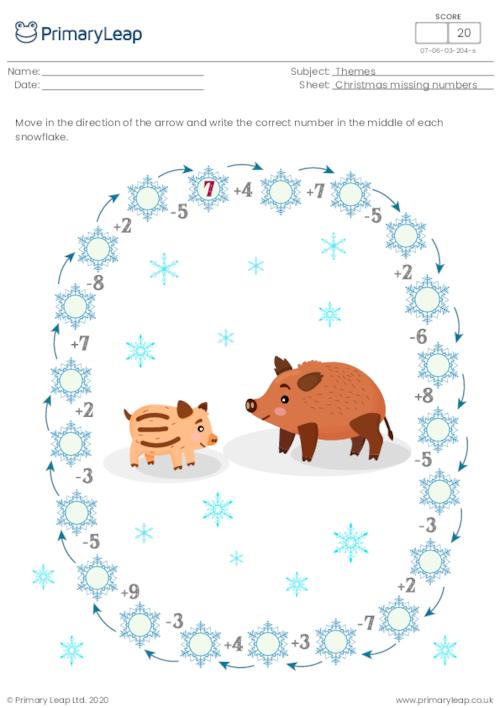### Christmas graphic dictation (reindeer)

Students start at the dot and follow the directions of the arrows to draw a picture (reindeer) on the grid. This Christmas themed resource can be used in the classroom or at home.

Age: 9 - 10
1
2
3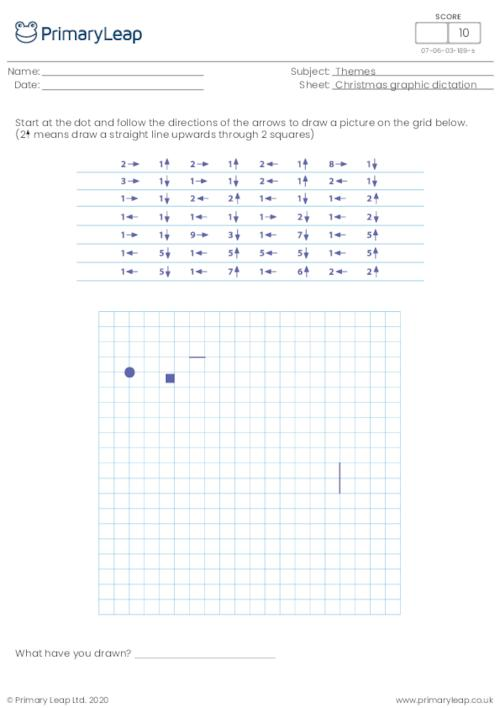### Christmas graphic dictation (candle)

Students start at the dot and follow the directions of the arrows to draw a picture on the grid.

Age: 9 - 10
1
2
3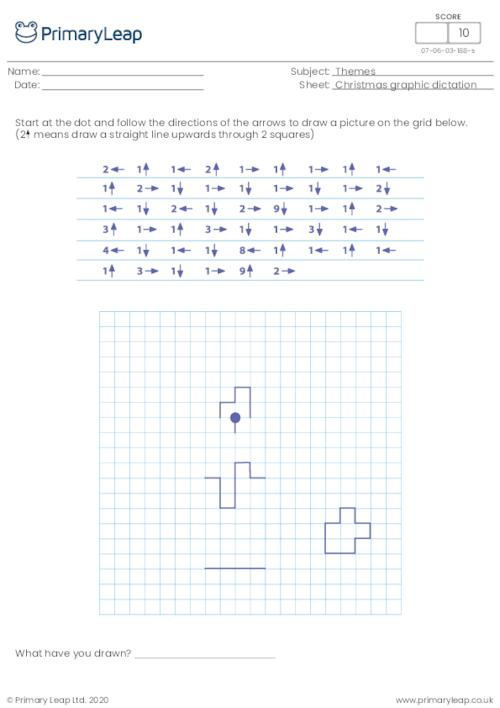### Halloween colour by number - Devil

This fun Halloween themed colour by number activity will help children recognise numbers 1 - 7.

Age: 9 - 10
1
2
3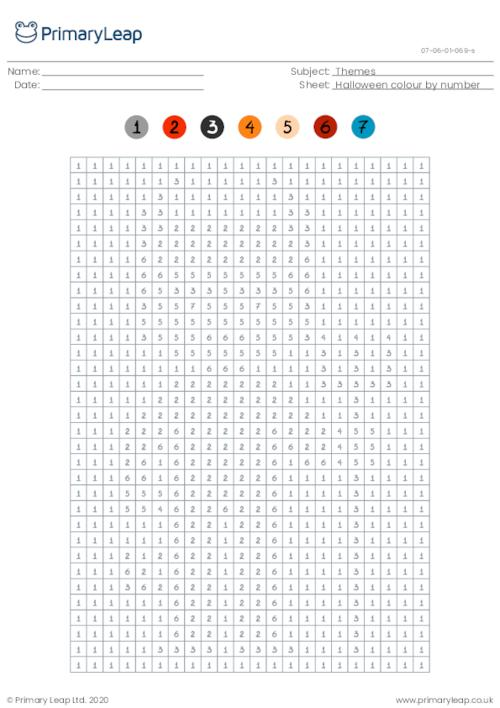### Translations

Translating s shape means sliding it up or down or side to side. You may not change the shape in any way. This worksheet asks students to translate each shape.

Age: 9 - 10
1
2
3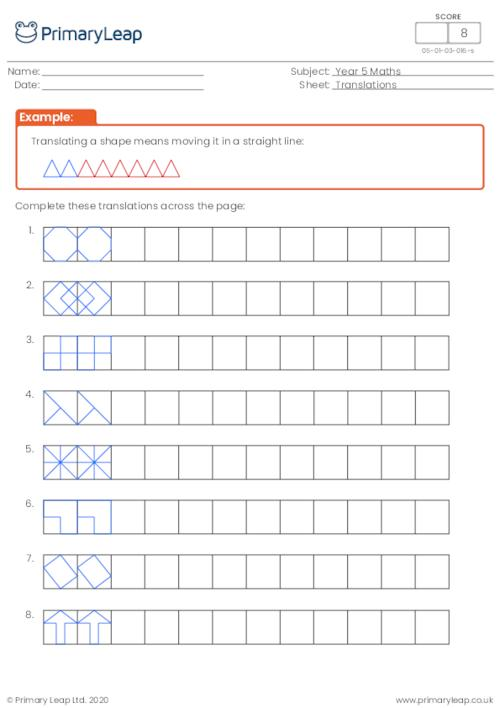### Properties of 3D shapes

This maths worksheet on 3D shapes asks students to complete the table by filling the missing properties.

Age: 9 - 10
1
2
3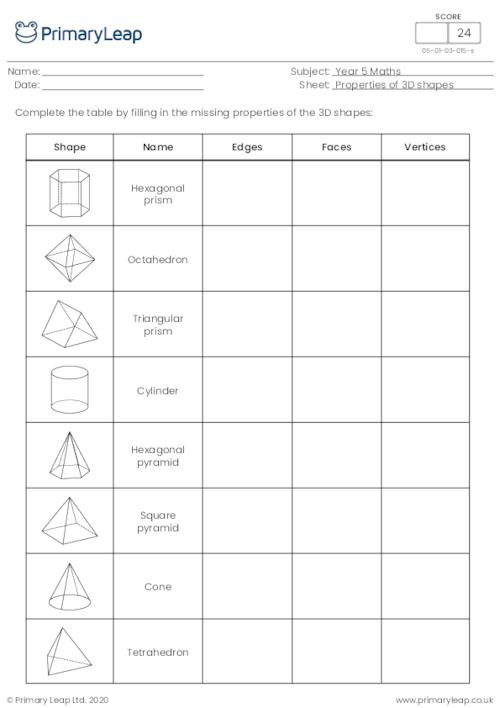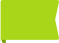Free

### 3D shape nets

Students match the correct net with the 3D shapes by completing the table.

Age: 9 - 10
1
2
3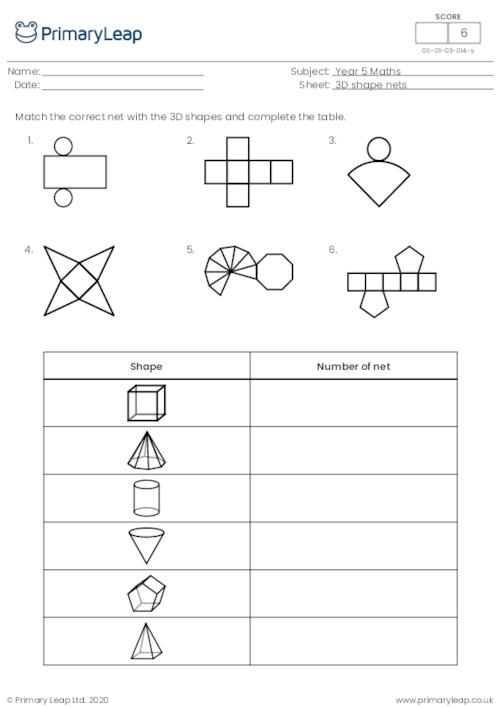### Naming 2D shapes

Students name the 2D shapes.

Age: 9 - 10
1
2
3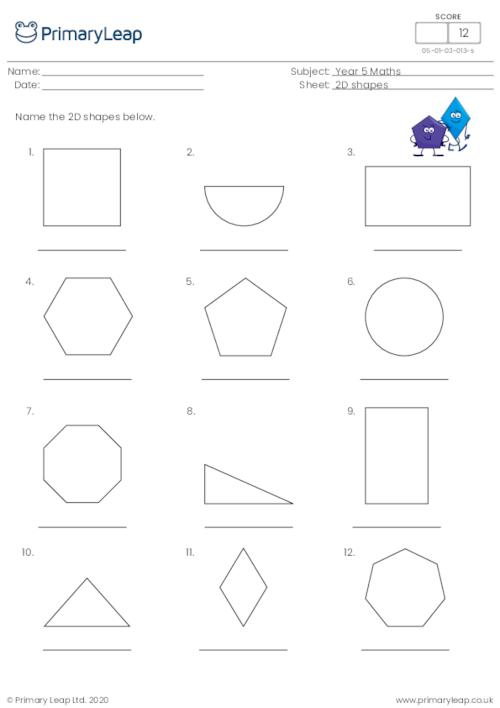### Classifying angles

Students label each angle with 'acute', 'obtuse' or 'reflex'.

Age: 9 - 10
1
2
3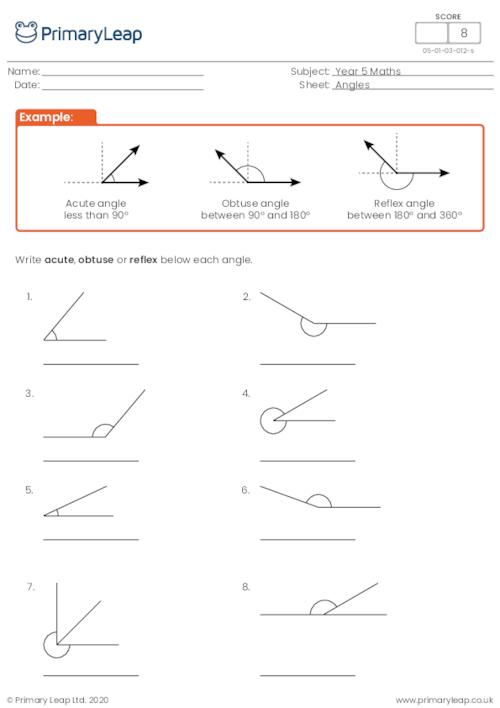### Regular and irregular shapes

Students are asked to tick the correct box to show whether the shape is regular or irregular.

Age: 9 - 10
1
2
3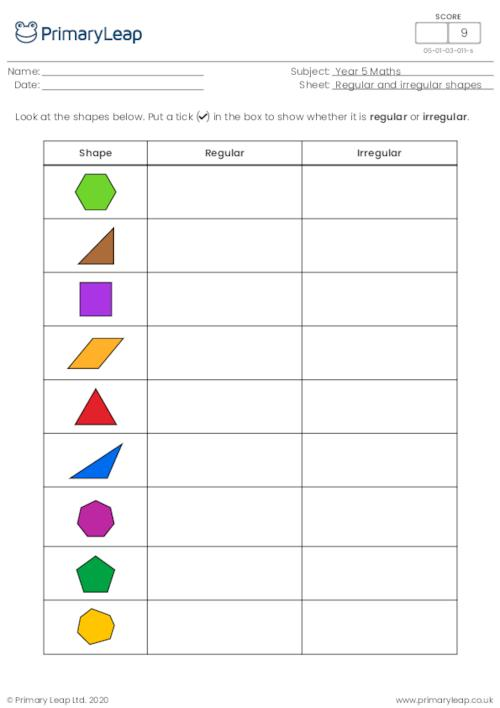### Estimating mass

Students estimate the correct weight of each object.

Age: 9 - 10
1
2
3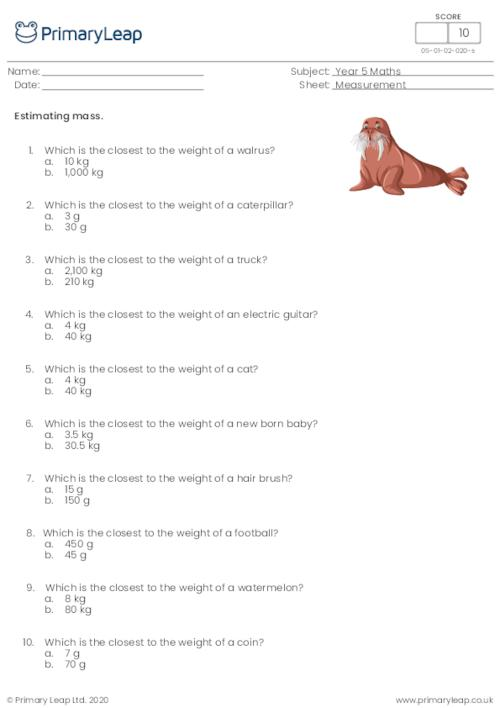Free

### Metric units of weight

Students are asked to choose the correct unit to measure the weight of different objects.

Age: 9 - 10
1
2
3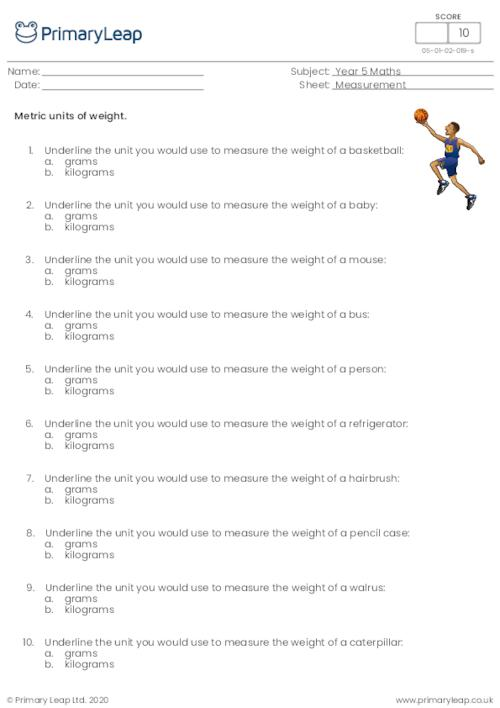### Converting units of length

Students read interesting facts and convert the different units of length.

Age: 9 - 10
1
2
3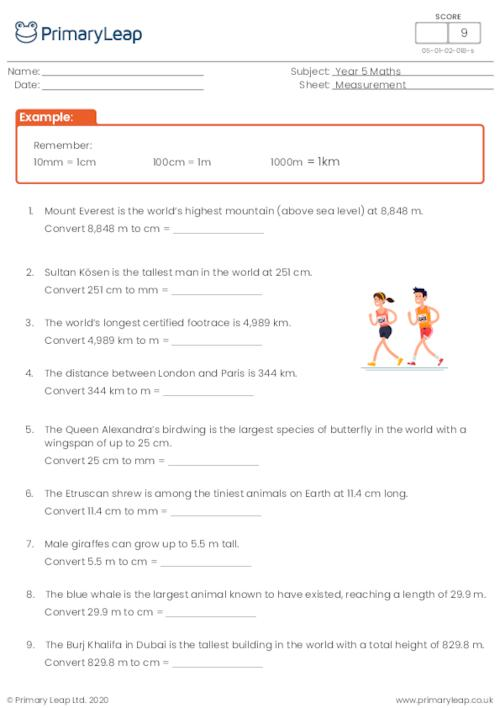### Metric units of length

Students are asked to choose the correct unit to measure different things.

Age: 9 - 10
1
2
3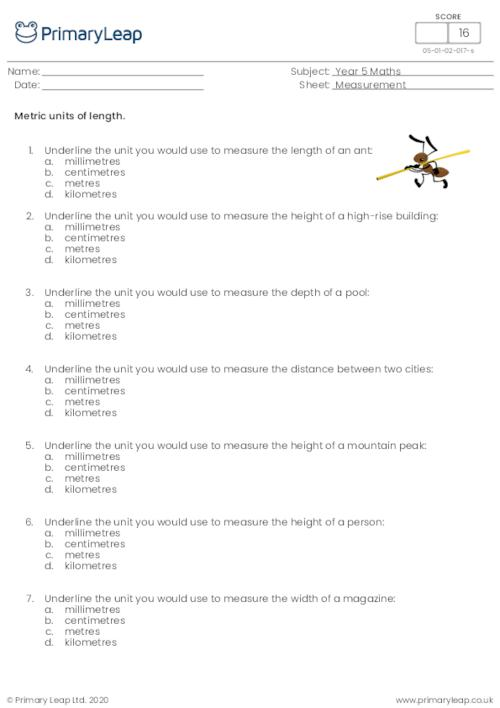### Area

Students complete the tables by filling in the missing measurements and then answer the questions.

Age: 9 - 10
1
2
3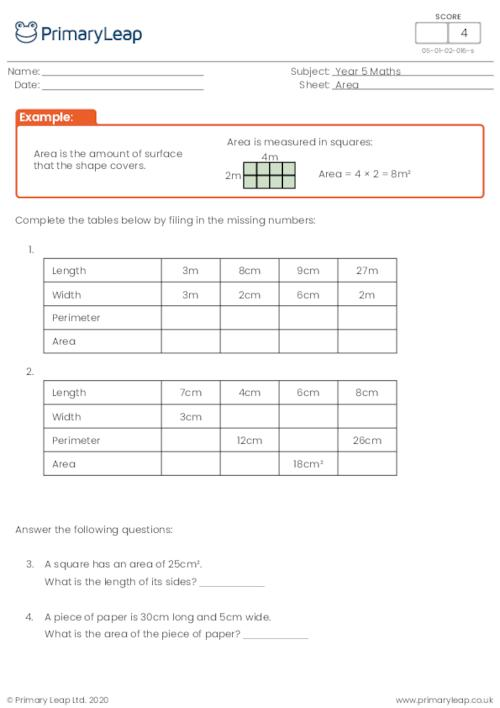### Finding the common factors of two numbers

Students are asked to find the common factors of two numbers.

Age: 9 - 10
1
2
3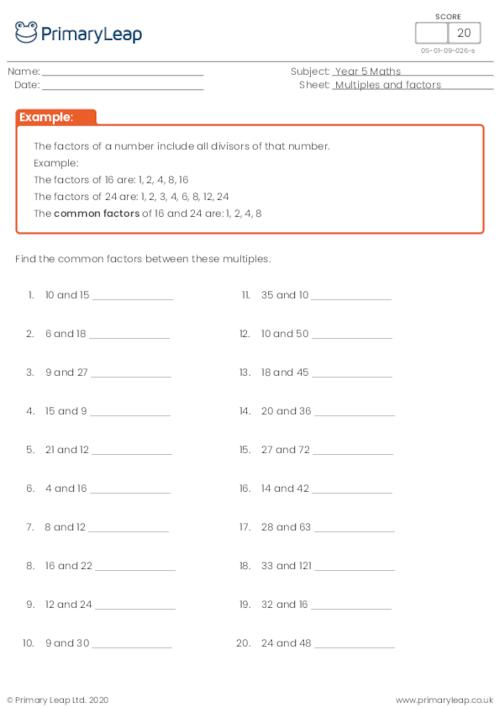### Finding the factor pairs of a number

Students are asked to find all the factor pairs of a number.

Age: 9 - 10
1
2
3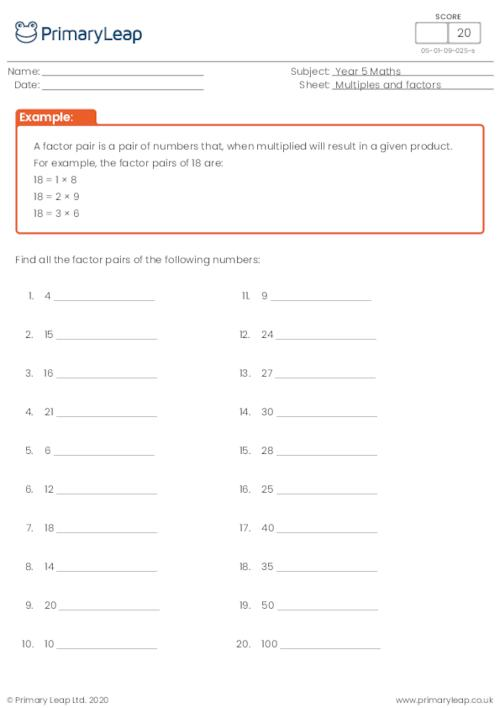### Identifying multiples and factors

Students identify the multiples and factors of each number sentence.

Age: 9 - 10
1
2
3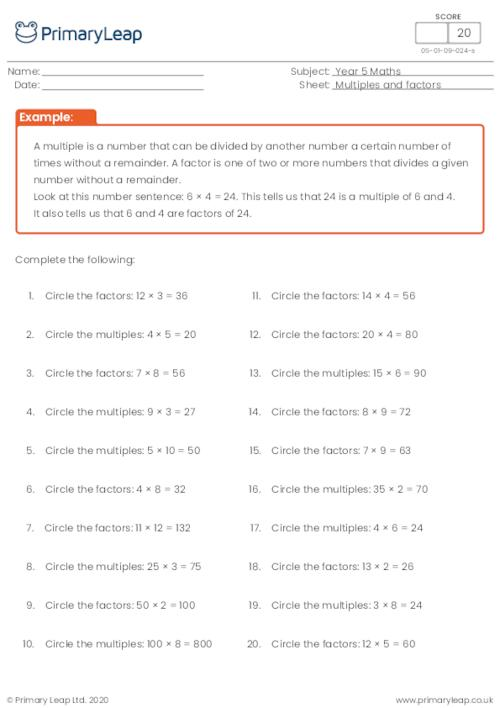### Recognising prime numbers up to 100

Students circle the prime numbers up to 100.

Age: 9 - 10
1
2
3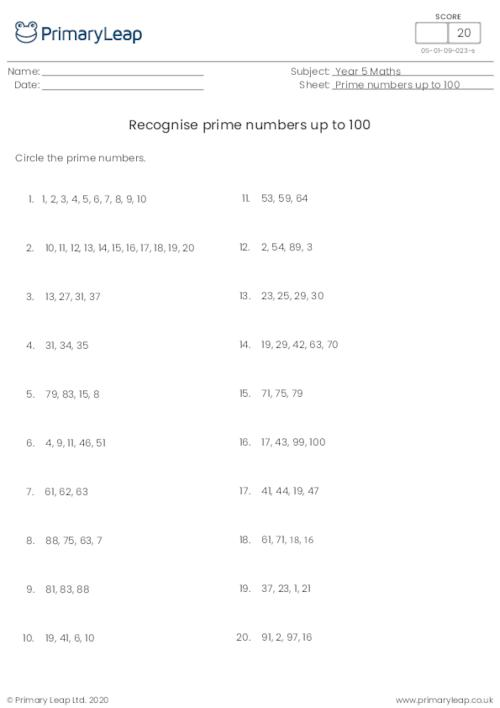### Writing prime numbers up to 20

Students practise writing the prime numbers up to 20.

Age: 9 - 10
1
2
3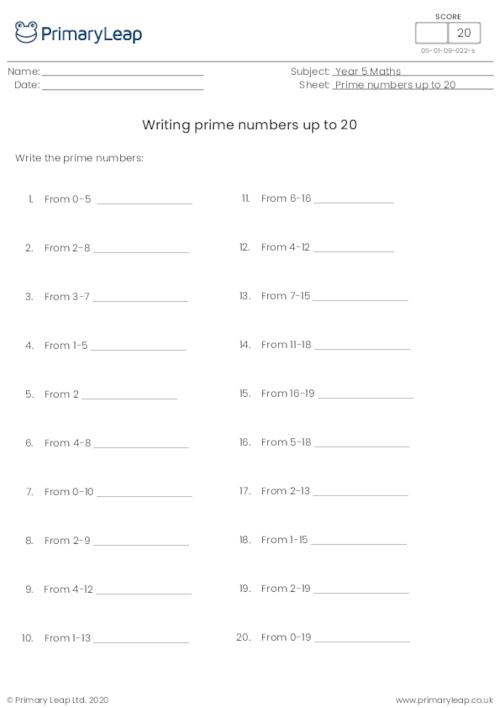### Recognising prime numbers up to 25

Students learn what a prime number is and recognise prime numbers up to 25.

Age: 9 - 10
1
2
3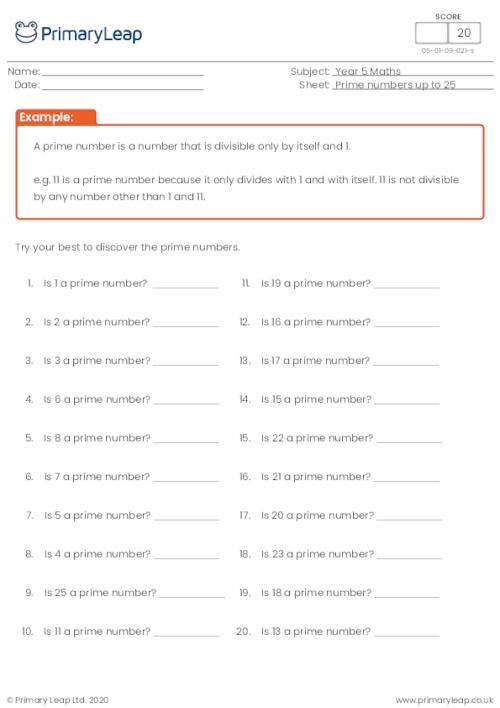### Recognising square numbers and cube numbers up to 12

Students practise finding the square of a number and the cube of a number without an example.

Age: 9 - 10
1
2
3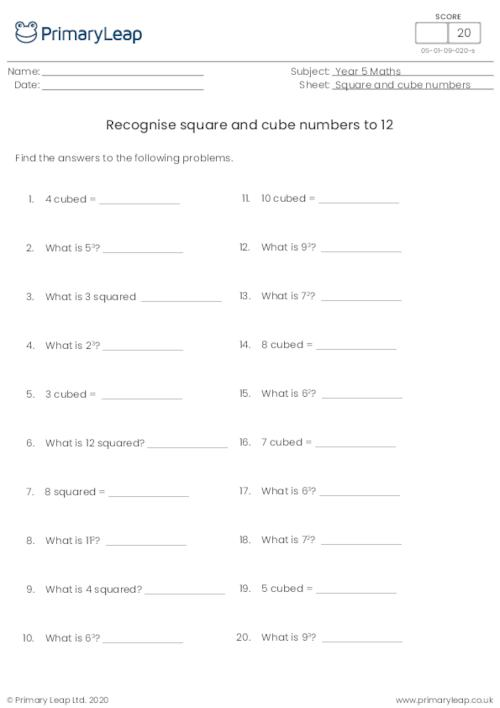### Recognising square numbers and cube numbers up to 12

Students practise finding the square of a number and the cube of a number.

Age: 9 - 10
1
2
3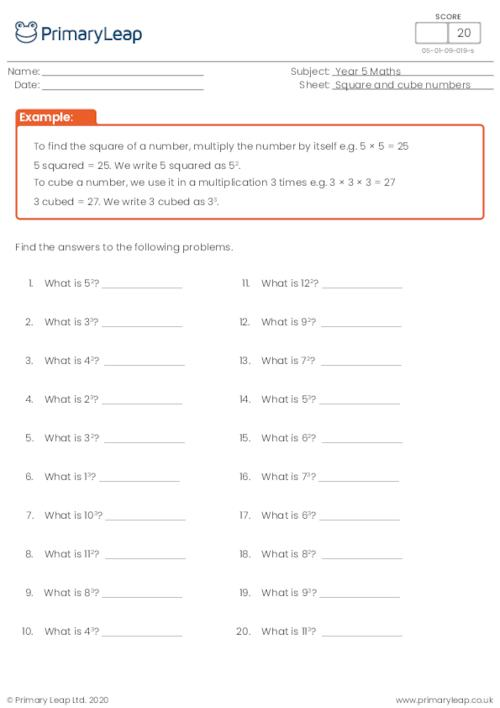### Recognising square numbers and cube numbers

Students learn how to square a number and how to cube a number.

Age: 9 - 10
1
2
3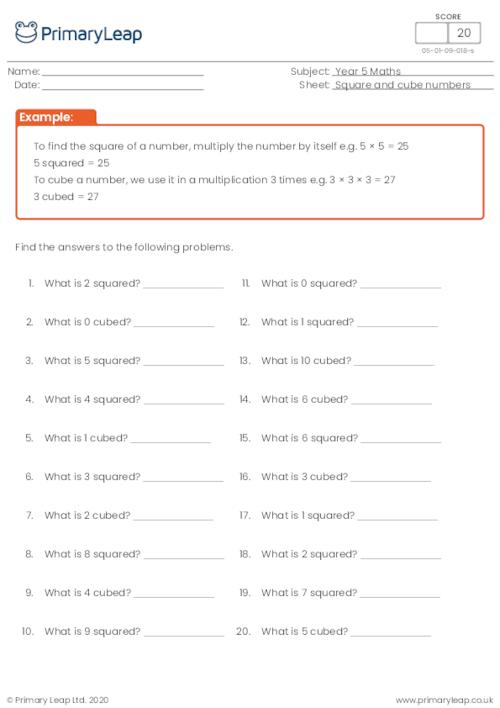### Multiply numbers up to 4 digits

Students are asked to multiply 4 digit numbers by a one or two-digit number.

Age: 9 - 10
1
2
3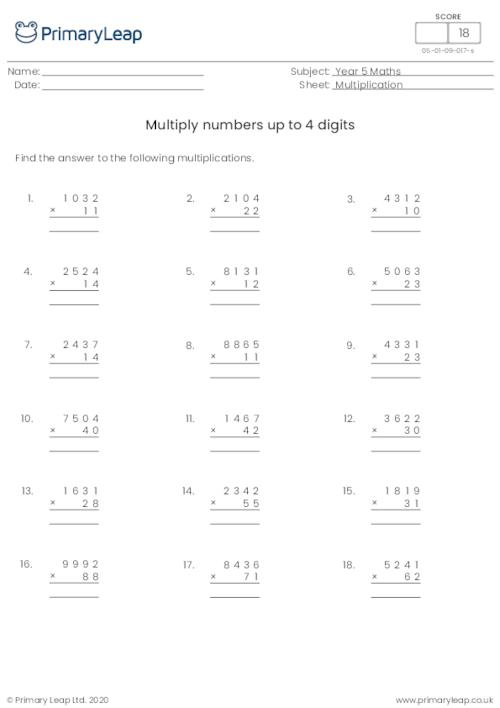### Multiply numbers up to 3 digits

Students practise multiplying 3 digit numbers by a 1 or 2 digit number.

Age: 9 - 10
1
2
3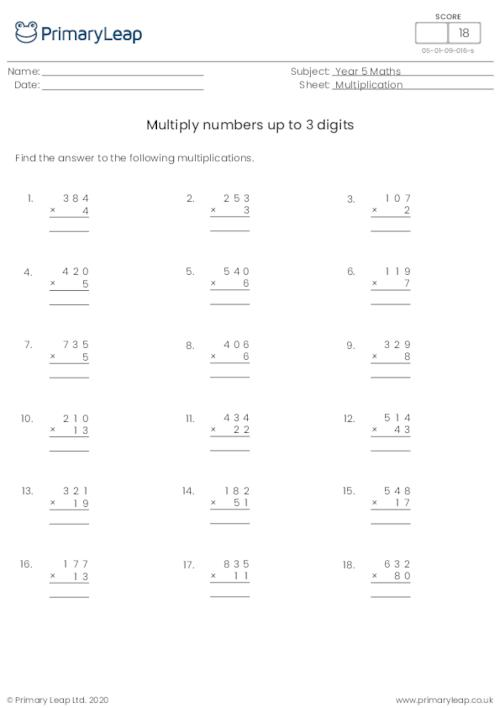### Multiply numbers up to 2 digits

Students practise multiplying numbers up to 2 digits.

Age: 9 - 10
1
2
3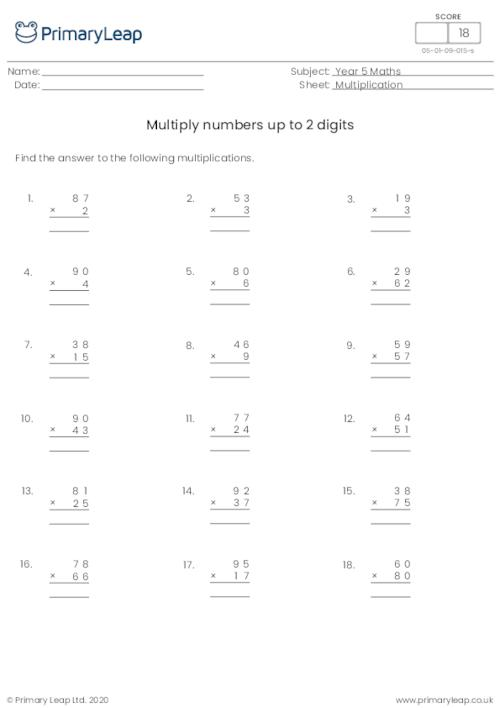### Multiply and divide decimals by 10, 100 and 1000

Students practise multiplying and dividing harder decimal numbers by 10, 100 and 1000.

Age: 9 - 10
1
2
3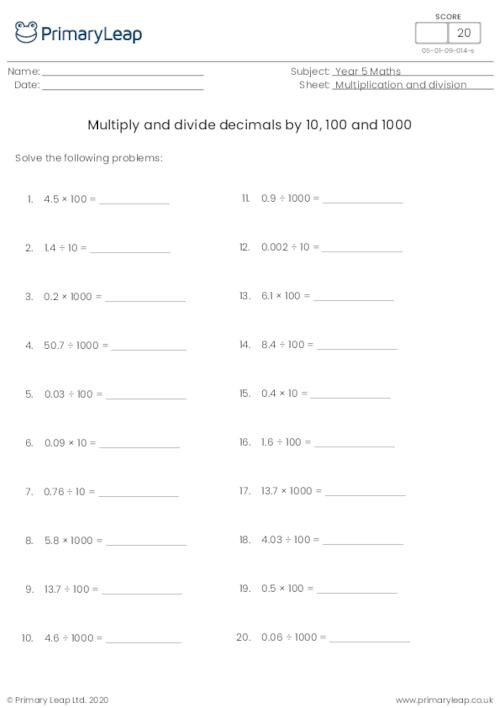### Multiply and divide decimals by 10, 100 and 1000

Students practise multiplying and dividing decimals by 10, 100 and 1000.

Age: 9 - 10
1
2
3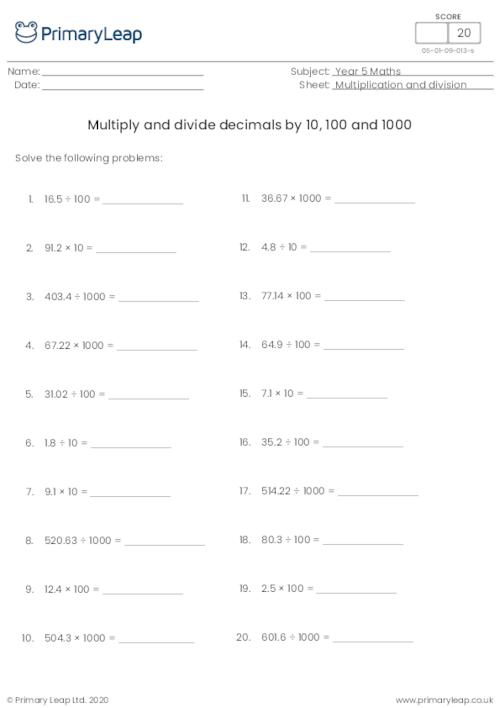### Multiply and divide decimals by 10, 100 and 1000

Students multiply and decimals by 10, 100 and 1000.

Age: 9 - 10
1
2
3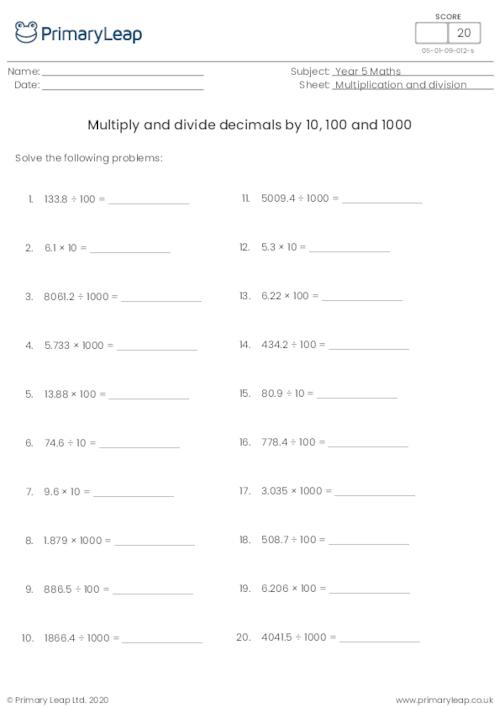### Dividing decimals by 10, 100 and 1000

Students practise dividing harder decimal numbers by 10, 100 and 1000.

Age: 9 - 10
1
2
3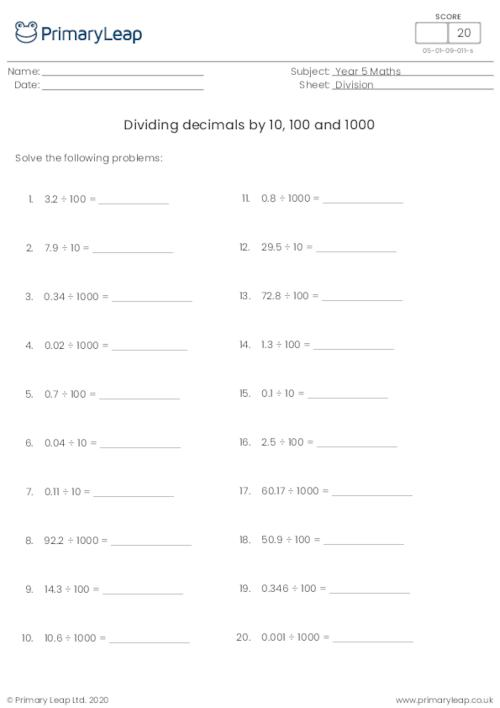### Dividing decimals by 10, 100 and 1000

Students practise dividing decimal numbers by 10, 100 and 1000.

Age: 9 - 10
1
2
3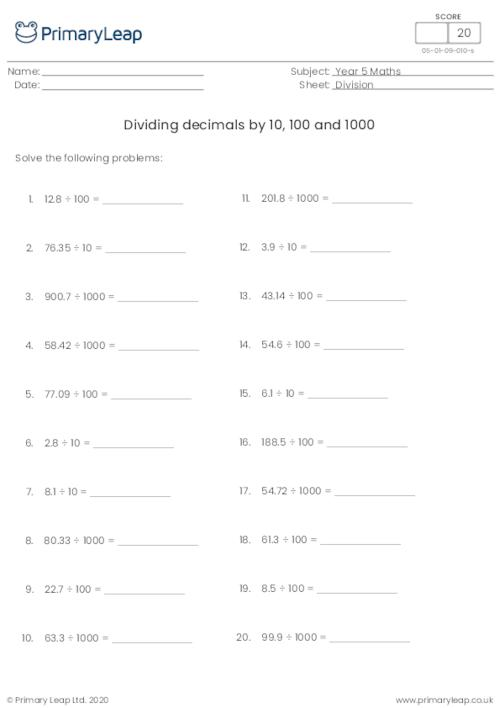### Dividing decimals by 10, 100 and 1000

Students practise dividing decimals by 10, 100 and 1000.

Age: 9 - 10
1
2
3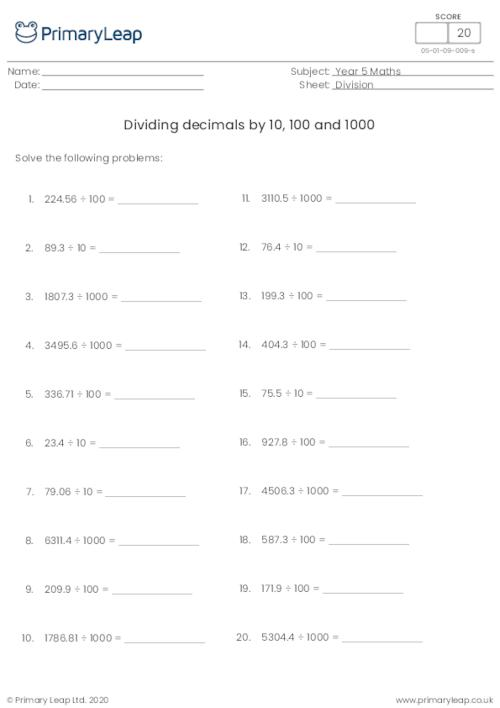### Dividing decimals by 1000

When dividing decimals by 1000, move the decimal point three spaces to the left. Students practise dividing decimals by 1000.

Age: 9 - 10
1
2
3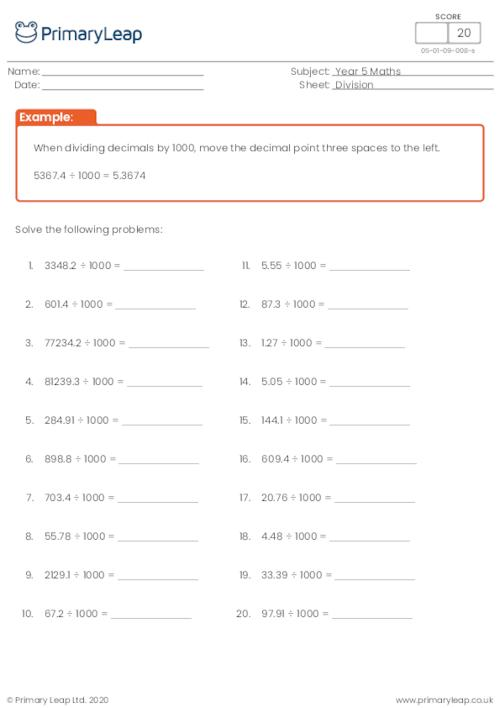### Dividing decimals by 100

When dividing decimals by 100, move the decimal point two spaces to the left. Students practise dividing decimals by 100.

Age: 9 - 10
1
2
3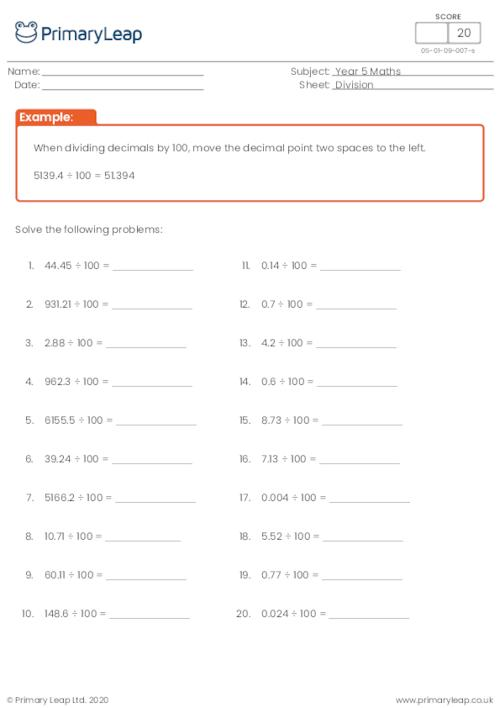### Dividing decimals by 10

When dividing decimals by 10, move the decimal point one space to the left. Students practise dividing decimal numbers by 10.

Age: 9 - 10
1
2
3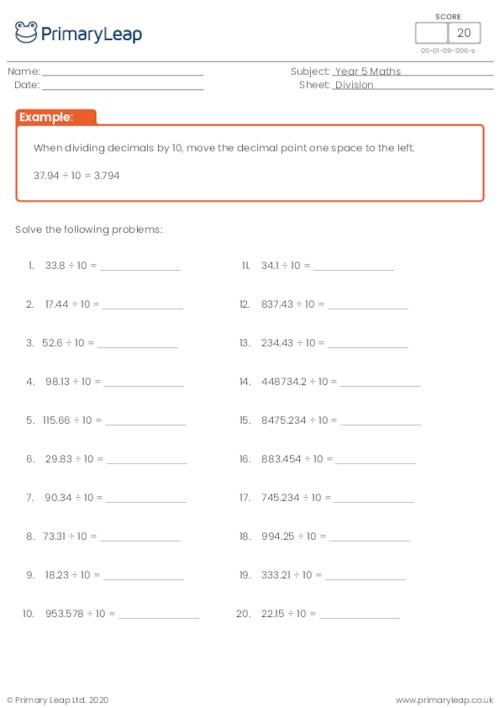### Multiply decimals by 10, 100 and 1000

Students practise multiplying decimals by 10, 100 and 1000 with harder numbers.

Age: 9 - 10
1
2
3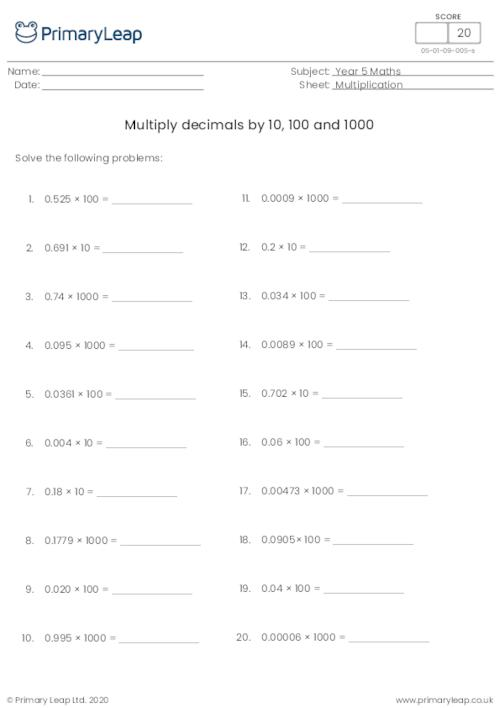### Multiply decimals by 10, 100 and 1000

This level 2 worksheet asks students to multiply the decimal numbers by 10, 100 and 1000.

Age: 9 - 10
1
2
3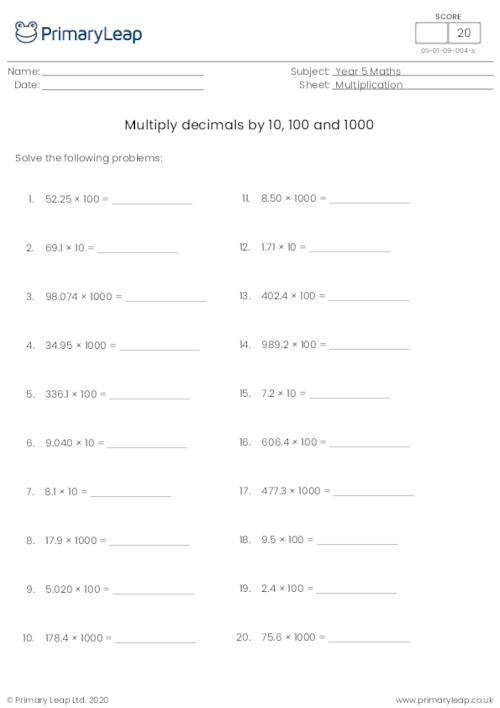### Multiply decimals by 10, 100 and 1000

Students practise multiplying decimals by 10, 100 and 1000.

Age: 9 - 10
1
2
3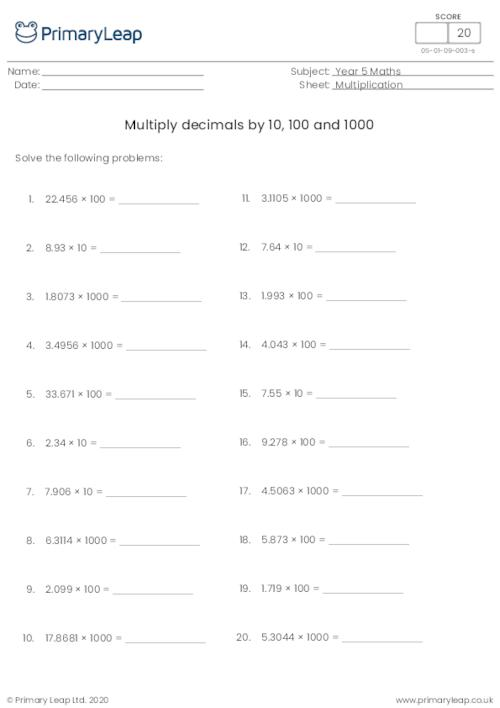### Multiply decimals by 1000

When multiplying decimals by 1000, move the decimal point three spaces to the right. Students practise multiplying decimals by 1000.

Age: 9 - 10
1
2
3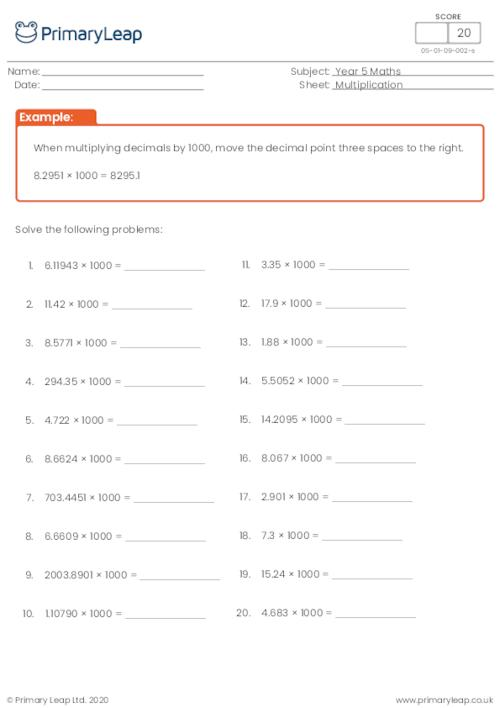### Multiply decimals by 100

When multiplying decimals by 100, move the decimal point two spaces to the right. Students practise multiplying decimal numbers by 100.

Age: 9 - 10
1
2
3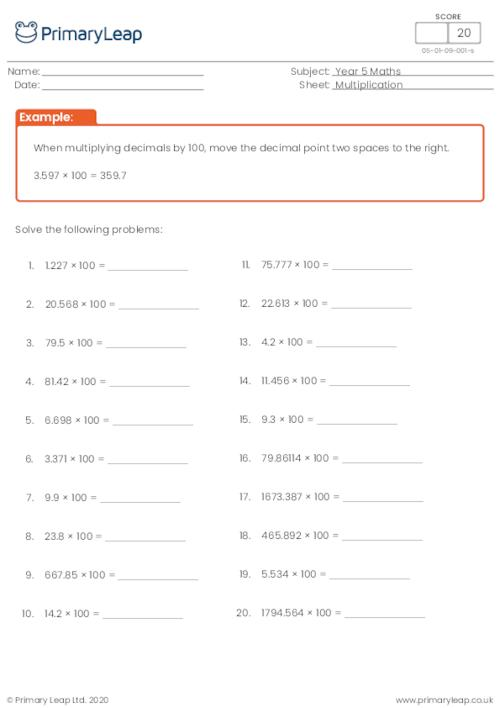### Multiply decimals by 10

When multiplying decimals by 10, move the decimal point one space to the right. Students are asked to multiply decimal numbers by 10.

Age: 9 - 10
1
2
3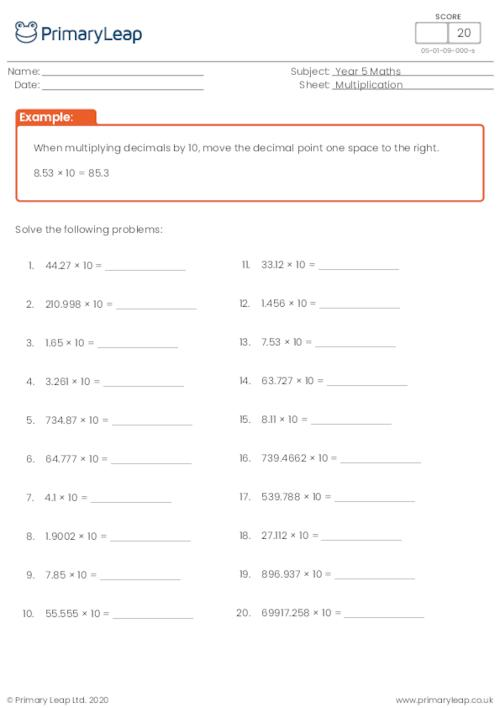### Converting decimals to fractions

Students convert 1 or 2 digit decimals to fractions.

Age: 9 - 10
1
2
3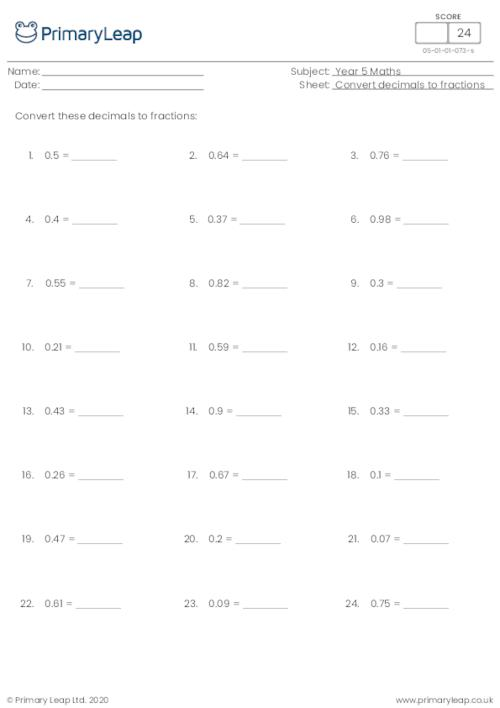### Converting fractions to decimals (denominator of 10 or 100)

Students practise converting fractions to decimals with a denominator of 10 or 100.

Age: 9 - 10
1
2
3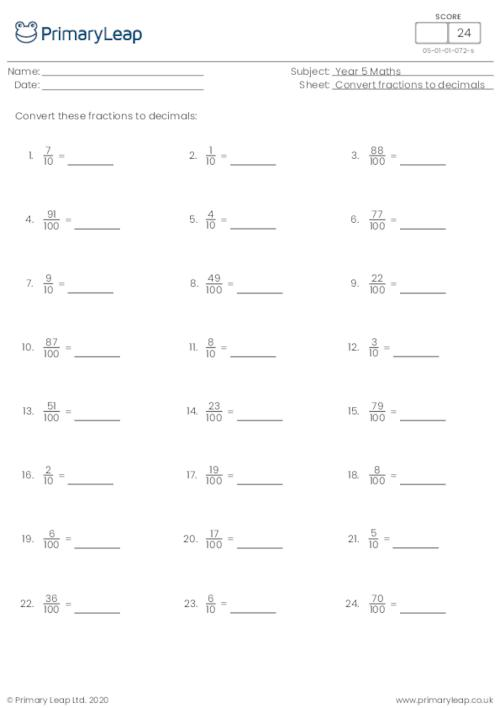### Converting fractions to decimals (denominator of 100)

Students practise converting fractions to decimals with a denominator of 100.

Age: 9 - 10
1
2
3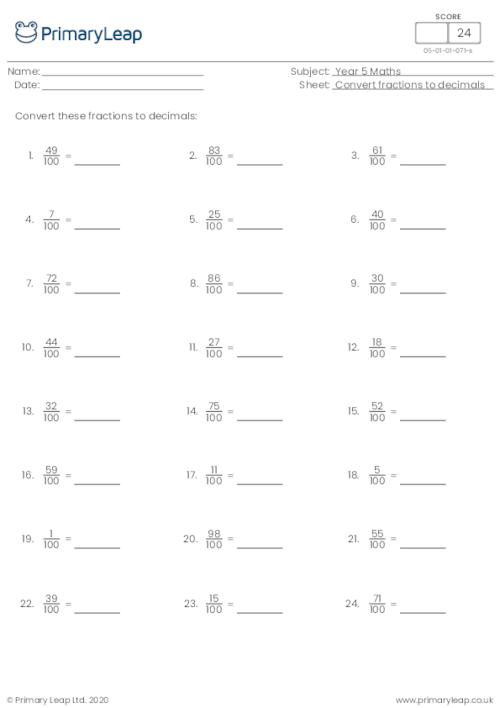of 5## Improve your child's mental and written calculation skills with our year 5 maths worksheets

In year 5, your child will continue to practise mental and written calculation methods and use all the operations to solve problems. Our fun and engaging maths worksheets will help your child to multiply fractions, learn more about decimals and percentages, round numbers up to 1,000,000, and much more.Learn

One of the best things about printable worksheets is that they make learning fun and easy. Explore our educational resources based on Year 5 Maths and keep your children learning through these fun and engaging exercises.Progress

Our Year 5 Maths printable worksheets are designed for progressive learning. Our engaging content enables a child to learn at their own speed and gain confidence quickly. When we help a child believe in themselves, we empower them for life.Improve

Printable worksheets are a great way to enhance creativity and improve a child’s knowledge. Help your child learn more about Year 5 Maths with this great selection of worksheets designed to help children succeed.